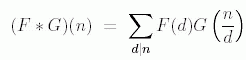ENG  RUS Timus Online JudgeOnline Judge
Задачи
Авторы
Соревнования
О системе
Часто задаваемые вопросы
Новости сайта
Форум
Ссылки
Архив задач
Отправить на проверку
Состояние проверки
Руководство
Регистрация
Исправить данные
Рейтинг авторов
Текущее соревнование
Расписание
Прошедшие соревнования
Правила

Novosibirsk SU contest. Petrozavodsk training camp. Summer 2007

Соревнование завершено

C. Multiplicative Functions

Ограничение времени: 2.0 секунды
Ограничение памяти: 64 МБ
In number theory, a multiplicative function is an arithmetic function F(n) of the positive integer n with property that F(1) = 1 and whenever a and b are coprime (gcd(a, b) = 1), then F(ab) = F(a)F(b).
The function E(n) defined by E(n) = 1 if n = 1 and = 0 if n > 1, is sometimes called multiplication unit for Dirichlet convolution or simply the unit function. If F and G are two multiplicative functions, one defines a new multiplicative function F * G, the Dirichlet convolution of F and G, bywhere the sum extends over all positive divisors d of n. With this operation, the set of all multiplicative functions turns into an abelian group; the identity element is E.
In this task you have to find the inverse of a multiplicative function. To cope with overflow problem, we define arithmetic functions as: F: N —> Z2007 where N is the set of positive integers, and Z2007 is a residue ring (ring of integers 0–2006, where arithmetic operations + and × are performed modulo 2007). Function G is called the inverse of function F if and only if F * G = G * F = E.
You are given the first N values of function F, you need to find the first N values of the inverse function G.

Исходные данные

In the first line of the input one number N is written (1 ≤ N ≤ 104). In the second line values F(1), F(2), F(3), …, F(N) are listed. Numbers are separated by spaces. (Each value is nonnegative and doesn't exceed 2006.)

Результат

In the first line of the output print first N values of inverse function G, separated by spaces: G(1), G(2), …, G(N).

Пример

исходные данныерезультат
16
1 1 1 1 1 1 1 1 1 1 1 1 1 1 1 1
1 2006 2006 0 2006 1 2006 0 0 1 2006 0 2006 1 1 0
Источник задачи: Novosibirsk SU Contest. Petrozavodsk training camp, September 2007
Чтобы отправить решение этой задачи на проверку перейдите в Архив задач: 1554. Multiplicative Functions